# LOG#067. SM(IX): Summary.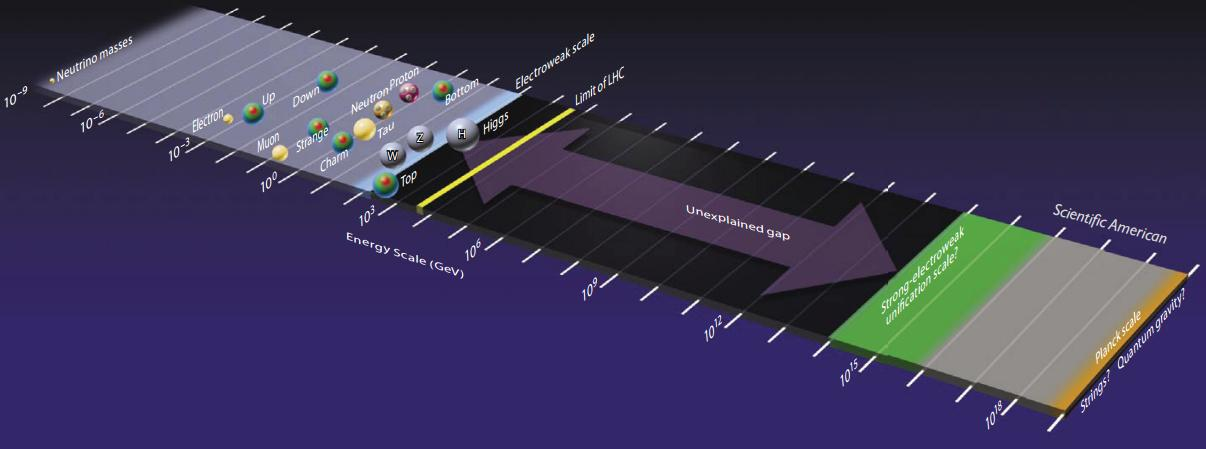What is the SM? What it does?What is not the SM? What it does not?

1) A local relativistic quantum field theory describing matter-energy and the electroweak and strong interactions up to a distance. It is a “correct”, “effective” (approximate) within known experimental errors and “simple” (in principle not in structure) theory describing almost everything with the exception of the the gravitational force, dark matter and dark energy.

2) After spontaneous symmetry breaking (SSB), the SM lagrangian breaks into: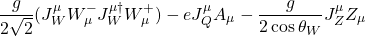3) The SM is a mathematically conistent renormalizable, Yang-Mills gauge field theory in 4 spacetime dimensions.

4) The SM predicts (not only fits) some phenomena tested in experiments. E.g.: the existence and form of the weak neutral currents (NC), the existence and mases of the W and Z bosons, the existence of the charm, the botton and the top quarks (for experts: the existence of such heavy quarks is vindicated the celebrated GIM mechanism).

5) Free parameters. Depending on how you count or select the free parameters for renormalization, they oscillate between 17 and 28 free parameters.

6) There is no explanation or prediction of the fermion masses, which vary over several orders of magnitude, or any of the CKM/PMNS mixing parameters. However, note that the mixing parematers are related to coupling constants rations and then, they are related to the ratios of the masses in the SM somehow, but we do not know how and why.

7) The SM includes but does NOT explain charge quantization: every particle has charges which are proportional to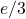to a high level of precision and accuracy.

8) The gauge structure in the SM is encoded in the gauge group. It has 3 separate gauge couplings.

9) The electroweak sector/piece of the SM is chiral and parity violating. It also breaks charge conjugation and CP symmetry as well.

10) There are 3 and only 3 families or generations. Two of them seems to be heavier copies of the first family. That is, if we set the fundamental or prime family as the one formed by:Then the remaining 2 generations are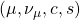and. The SM gives NO explanation of why the additional 2 generations exist, and of course, the SM gives no explanation of any of the structure and number of these and further families.

11) Higgs particles/bosons. The minimal SM predicts an elementary Higgs field to generate fermion masses and the gauge boson masses for the W and Z bosons. The Higgs particle mass should not be too different from the W or Z mass for the total SM consistency, i.e., the SM predicts that. Theoretical arguments could suggest the existence of low energy SUSY in order to explain how the Higgs mass avoids to receive large renormalizations to its mass. This argument uses cancellation or interference between Feynman diagrams. However, in spite of the fact the Higgs have been discovered this year (2012), we have not found evidence for supersymmetry (SUSY) yet. A broken high-energy SUSY looses some of the appealing for explaining the low Higgs mass and/or solving the hierarchy problem between the electroweak scale and the Planck scale.

12) The existence of generations, the structure of masses and mixing parameters, both in the quark and lepton sectors, suggest the existence of additional “flavor symmetries”: they can be “horizontal” local gauge symmetries or global discrete flavor symmetries.

13) The complex structure of the gauge sector in the SM and complementary experimental and theoretical evidences suggest that the local gauge symmetry group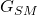should be embedded into a larger gauge group. It is called the Grand Unification, and theories like these providing an initial gauge group are called Grand Unified Theories or GUT. They would be truly unified theories of electroweak and strong interactions and, likely, the Theory Of Everything (TOE) if we include the gravity into the gauge framework. However, and unfortunately, gravity is NOT a (at least normal) gauge theory. Some particular elections of GUT groups likeare well known in the literature and they do have some predictions that can be tested in the LHC and hopefully additional experiments/observations.

14) Axions and the strong CP problem. Currently, the QCD sector does NOT allow for pure CP violations. However, on theoretical arguments, the SM lagrangian can be complemented with the so-called theta term piece, a pure QCD CP violating lagrangian piece: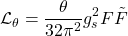and where we have defined the dual field strength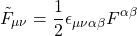The theta term breaks the P, T and CP symmetries in the QCD sector. Of course, CP symmetry in the QCD sector can be measured experimentally. This term, if it were proved to exist, it would be very tiny sinceand it would induce an electric dipole moment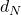to the neutron. The rather stringent limits on the dipole moment provide the previous bound. The smallness of this parameter is called the strong-CP problem and one simple solution (but it is not easy to test at all) is the so-called Peccei-Quinn mechanism and the existence of an extra and additional global symmetry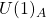imposed by the theory in such a way thatbecomes a dynamical variable rather than a constant and it would have a zero value at the minimum of the (Higgs) potential. It also implies the existence of a new (pseudo)scalar particle called the axion. Laboratory, astrophysical and cosmological tests have constrained only a very small window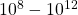GeV for the Peccei-Quinn scale or the ultralow energy(mass) axion for the axion mass scale (microGeV or even lower) at which thesymmetry is broken.

15) The SM and gravity are unrelated. Gravity is not fundamentally unified with the electroweak and strong interactions in the SM. In fact, there is no quantum theory of gravity at current time, only some candidates and temptative (highly speculative) theories.

16) The cosmological constant in the Eisntein’s field equations for gravity can be thought as a vacuum energy. The vacuum expectation value of any scalar Higgs-like field generates indeed a cosmological constant: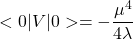when we evaluate such a quantity at the minimum of the potential. It has a large value when the theory couples to gravity due to the fact that a constant energy density IS EQUIVALENT to a cosmological constant. The cosmological constant can be written then as: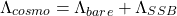and whereis the primordial cosmological constant ( the value of the vacuum energy in the absence of SSB) and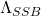is the term or cosmological constant generated after SSB by the own Higgs mechanism. We have, in the most optimistic scenario, that: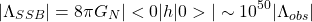It is about 50 or 60 orders of magnitude bigger than the observed cosmological constant (coming from  cosmological observations). This is the biggest problem in theoretical physics and likely one of the worst “predictions” of any theory. It remind us the infamous ultraviolet catastrophe in the XIX century though. We hope to solve this formidable problem in the near future somehow. Technically, we could solve the problem naively by adding a new extra termto V, in such a way that V were equal to zero at the minimum. However, such a naive procedure fails. With our current knowledge of the symmetries of Nature, there is no reason to relate the bare cosmological constant and the SSB cosmological constant. That is, the energy density induced by Higgs potential after SSB is many, many orders of magnitude larger than the experimental upper limit. This is called the cosmological constant problem.

Some solutions to this hard problem involve:

i) Using Kaluza-Klein theories in 5 or higher dimensions.

ii) Supergravity theories. These theories of local supersymmetry including gravity solve partially the problem. They can solve the cosmological constant problem but they don’t explain what is the theory of quantum gravity or even we ignore yet if supergravity (SUGRA) theories are renormalizable! Therefore, in current time, they don’t provide any obvious solution to the cosmological constant problem at the fundamental level (it goes beyond the numerical values, as the previous explanations show).

iii) Superstring theory/M-theory/Brane-worlds. They are a wide class of theories that unify gravity and the remaining interactions. It may yield to finite (renormalization free) theories of gravity and quantum gravity or every fundamental interaction. It is not clear yet if they can solve the cosmological constant problem at all!

17) The SM does not say what Dark Matter/Dark Energy are and/or what are they made of. We do know they are stuff we can not explain with the SM. In fact, it seems that the observable Universe that we do understand is at most a ridiculous 5% of the whole Universe. It is puzzling and pushing to go beyond the Standard Model: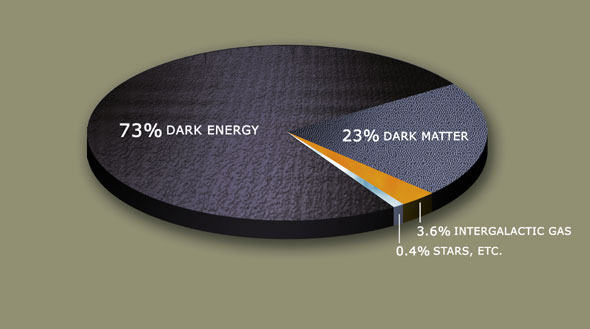18) The origin of the Higgs mass value is a free parameter in the SM, and too, its couplings to the fermions. Then, the origin of the Higgs coupling to fermions is also unknown. Then, the SM can not explain the origin of mass at fundamental level.

19) The Universe is likely made of matter mainly. The SM does not explain why we don’t observe antimatter in the same proportion. This is sometimes called the antimatter problem or the baryon asymmetry problem.

20) The SM predicts a null mass for neutrinos. The fact that neutrinos oscillate was one of the first experimental evidences, added to Dark Matter/Dark Energy and other phenomena, that the SM is not the whole story. The structure of neutrino oscillations via the PMNS matrix is essentially the opposite to the quark mixing. It seems that neutrino oscillations happen with maximal or almost maximal mixing, while the quark mixing happens with almost null mixing (or very soft mixing). We can not understand these mixing patterns in the SM.

21) The cosmic ray enigma. Cosmic rays hit Earth and produce particles we can detect with modern detectors. The cosmic ray energy primaries or the origin of the particle cascades we observe is a complete mystery, but we do know they have an incredible energy, PeV or higher! What is the mechanism of production of cosmic rays? What are primary cosmic rays? We can not know yet but we have some cool experiments working on that issue.

22) The neutrino is the lightest particle but, how many TOTAL neutrino species do exist? Experimentally we do know that there are 3 light neutrino species. But cosmological measurements allow for a little higher number of neutrino species. Is there a sterile neutrino? Could it be causing some of the anomalies we observe in DM and neutrino detection experiments? Is the neutrino a Majorana particle?23) Is the renormalization procedure necessary? Renormalization has been imposed as a physical requirement but we don’t understand if Nature does renormalization or if renormalization is a mere tool to provide finite answers.

And there are many other questions, some quite technical, that I will not review here, unless you consider them important. Let me know if you know some additional and interesting SM feature/issue or enigma…

Let the SM be with you!

This site uses Akismet to reduce spam. Learn how your comment data is processed.